Courses

# Test: Symmetry

## 10 Questions MCQ Test Mathematics (Maths) Class 6 | Test: Symmetry

Description
This mock test of Test: Symmetry for Class 6 helps you for every Class 6 entrance exam. This contains 10 Multiple Choice Questions for Class 6 Test: Symmetry (mcq) to study with solutions a complete question bank. The solved questions answers in this Test: Symmetry quiz give you a good mix of easy questions and tough questions. Class 6 students definitely take this Test: Symmetry exercise for a better result in the exam. You can find other Test: Symmetry extra questions, long questions & short questions for Class 6 on EduRev as well by searching above.
QUESTION: 1

### How many lines of symmetries are there in an isosceles triangle?

Solution: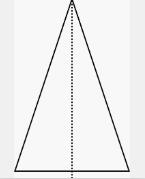An isosceles triangle has exactly two sides of equal length it has just 1 line of symmetry that runs from the vertex between the two sides of equal length to the midpoint of the side opposite that vertex.

QUESTION: 2

### How many lines of symmetries are there in an equilateral triangle?

Solution: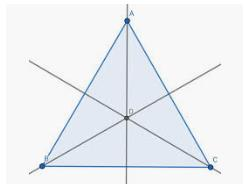An equilateral triangle has 3 lines of symmetry

QUESTION: 3

### A parallelogram has ______ lines of symmetry:

Solution:

A general parallelogram has no lines of symmetry. Some special ones (rhombus, rectangle, square) have lines of symmetry.

QUESTION: 4

The mirror image of ‘W’, when the mirror is placed vertically:

Solution:

In case of standard form of mirror image i.e., when the mirror is placed vertically, the object gets laterally inverted. In order words, the Left Hand Side (LHS) and Right Hand Side (RHS) of an object interchange their places while top and button remain the same.

QUESTION: 5

How many lines of symmetries are there in a square?

Solution: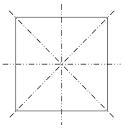For the square, it can be folded in half over either diagonal, the horizontal segment which cuts the square in half, or the vertical segment which cuts the square in half. So the square has four lines of symmetry.

QUESTION: 6

How many lines of symmetries are there in a rhombus?

Solution: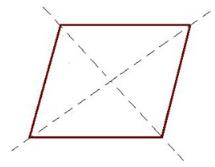Lines of symmetry in a rhombus = 2

A rhombus has two lines of symmetry that run through one vertex to the one directly across it. Unlike a square, planes through the center of each edge of a rhombus do not yield mirrored sides.

QUESTION: 7

Which of the following letters have reflection line of symmetry about vertical mirror?

Solution:

C and B has Horizontal line of symmetry ,Q has no line of symmetry and V has vertical line of symmetry .

QUESTION: 8

Which of the following alphabets has line symmetry?

Solution:

If you draw a line between A you see both the sides equal.

QUESTION: 9

The number of lines of symmetry a rectangle does have:

Solution: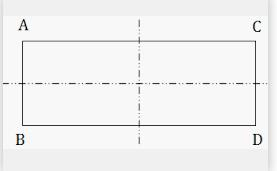Rectangle have 2 lines of symmetry

QUESTION: 10

The order of the rotational symmetry of the parallelogram about the centre is

Solution:

A general parallelogram has no lines of symmetry.